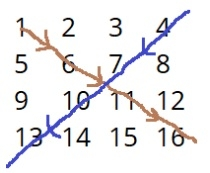# Print matrix in diagonal pattern

Given a 2d array of n*n and the task is to find the antispiral arrangement of the given matrix

Input : arr={1,2,3,4,
5,6,7,8,
9,10,11,12
13,14,15,16}
Output : 1 6 11 16 4 7 10 13## Algorithm

START
Step 1 -> declare start variables as r=4, c=4, i and j
Step 2 -> initialize array as mat[r][c] with elements
Step 3 -> Loop For i=0 and i<r and i++
Print mat[i][j]
Step 4 -> print
Step 5 -> Loop For i=0 and i<r and i++
Print mat[i][4-1-i]
End
STOP

## Example

#include<iostream>
#include <bits/stdc++.h>
using namespace std;
int main() {
int R=4,C=4,i,j;
int mat[R][C] = { {1,2,3, 4}, {5,6,7,8},{9,10,11,12},{13,14,15,16}};
for(i=0;i<R;i++) {
cout<<mat[i][i]<<" ";
}
cout<<"";
for(i=0;i<R;i++) {
cout<<mat[i][4-1-i]<<" ";
}
}

## Output

if we run the above program then it will generate the following output

1 6 11 16
4 7 10 13